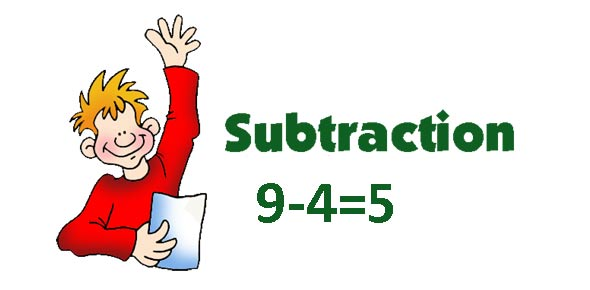# Operations - Addition And Subtraction

26 Questions | Total Attempts: 49SettingsRelated Topics
• 1.
5+0 =
• 2.
9+5 =
• 3.
1+2 =
• 4.
6+2 =
• 5.
5+1 =
• 6.
8+1 =
• 7.
0+6 =
• 8.
8+0 =
• 9.
2+8 =
• 10.
8+4 =
• 11.
1+1 =
• 12.
7+4 =
• 13.
2+0 =
• 14.
3+4 =
• 15.
6+3 =
• 16.
1+8 =
• 17.
7+1 =
• 18.
9+6 =
• 19.
1 + 1 =
• A.

4

• B.

3

• C.

1

• D.

2

• 20.
What color word is spelled right?
• A.

Blue

• B.

Blu

• C.

Blo

• D.

Bu

• 21.
What color word is spelled right?
• A.

Lello

• B.

Yellow

• C.

Yelo

• D.

Yelu

• 22.
What color word is spelled right?
• A.

Rab

• B.

Der

• C.

Red

• D.

• 23.
What color word is spelled right?
• A.

Blak

• B.

Back

• C.

Bluk

• D.

Black

• 24.
What color word is spelled right?
• A.

Fidn

• B.

Find

• C.

Ifnd

• D.

Dnif

• 25.